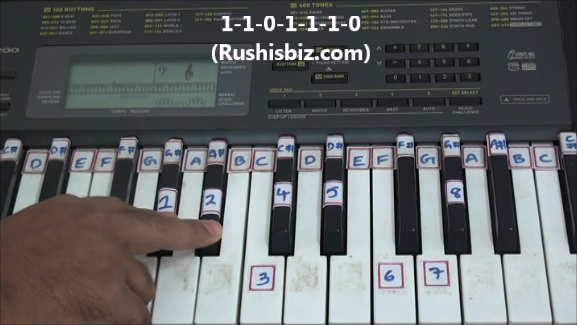HINDI - A B C D E F G H I J K L M N O P Q R S T U V W X Y Z

TAMIL - A B C D E F G H I J K L M N O P Q R S T U V W X Y Z

TELUGU - A B C D E F G H I J K L M N O P Q R S T U V W X Y Z

# “G#/Ab” Major Scale Positions – Piano Tutorials

Notes:  G#-1, A#-2, C-3, C#-4, D#-5, F-6, G-7, G#-8

Since it is a ‘G#’ major scale obviously ‘G#’ will be first note, how to find the remaining notes ?

The simple formula which is used for any major scale:

One- One- Zero- One-One- One-Zero  (1-1-0-1-1-1-0)

What do you mean by Ones and Zeros ?

One’s (whole step) – If there is a one key between two notes, then the interval of those two notes is One.

Eg: from G# to A# the interval is “one key”

Zero’s (half step) – If there are no keys or zero keys between two notes, then the interval of those two notes is Zero

Eg: from C to C# the interval is “zero” (Immediate keys)‘G#’ Major Scale Positions – Video Tutorials